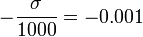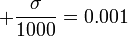# Difference between revisions of "Talk:2311: Confidence Interval"

Actually, if you integrate a normal distribution$\mathcal{N}(0,1)$ from$-\frac{\sigma}{1000}=-0.001$ to$+\frac{\sigma}{1000}=0.001$, you'll get a range of about 0.08% of all values. This would be bad because it would mean that, as big as the confidence interval appears in the picture, the more meaningful 1- or 3-sigma interval (whose size represents the uncertainty of the model) would be larger by a factor of 1250 or 3750, respectively. --Koveras (talk) 08:38, 26 May 2020 (UTC)